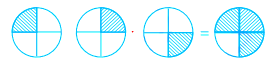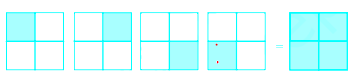# Fractions and Decimals worksheet for Class 7

Question 1.
Fill in the blanks: -
i. A fraction is a number which can be written in the form a/b, where a, b are   _____numbers and b ≠ 0.
ii. If numerator and denominator of a fraction have no common factor other than 1, then the fraction is said to be in its________________ form.
iii. if $\frac {c}{d} =\frac {m \times a}{m \times b}$ ,then fractions $\frac {a}{b}$ and $\frac {c}{d}$  are called_____________ fractions because they represent the _________portion of the whole.
iv. The value of the product of two proper fractions is__________ than each of the two fractions.
v. The product of two improper fractions is__________________ than the two fractions.
vi. A fraction whose denominator is any of the numbers 10,000, 1000 etc, called a____________
vii. 11.5 ÷ _______________ =1.
viii. If 312.5 ÷ 25 = 12.5, then value of 3.125 ÷ 25 is_____________.
ix. The product of a proper and an improper fraction is____________ than the improper fraction and __________then the proper fraction.
x. When a decimal number is multiplied by 10,000 or 1000 the digits in the product are same as in the decimal number but the decimal point in the product is shifted to the ___________by as, many of places as there are zeros over one.

Question 2.
State true / false.
i. A decimal fraction is a fraction where the denominator is 10 or higher power of 10.
ii. Every integer can be expressed as a fraction.
iii. Like decimals have the same number of decimal places.
iv. Natural numbers can be expressed as a proper fraction.
v. The reciprocal of $\frac {2}{7}$ is $\frac {7}{2}$
vi. The reciprocal of a proper fraction is a proper fraction.
vii. The reciprocal of an improper fraction is an improper fraction
viii. The product of 7 and $3 \frac { 6}{4}$ is $31 \frac {1}{2}$

Question 3
Convert 6.432 into a decimal fraction.

Question 4.
If 12 inches = 1 foot (ft) then change 549 inches into ft.

Question 5.
A fruit seller buys 712 fruits, of which 3/4 are apples. Of all the apples that he bought, 1/3 were found to be rotten. If he sold all the good apples at Rs. $5 \frac {1}{4}$ each. How much money did he receive on selling all the good apples.

Question 6.
If 21.875 litres of oil is in $3 \frac {1}{2}$ cans of tin of equal capacity, find the capacity of oil in each can.

Question 7
$\frac {1}{4 \frac {2}{7}} + \frac {1}{3 \frac {11}{13}} + \frac {1}{ \frac {5}{9}}$

Question 8
$\frac { 2\frac {1}{2} + \frac {1}{5}}{2\frac {1}{2} \div \frac {1}{5}}$

Question 9
The picture interpretsa. $3 \times \frac {1}{4}$ b. $3 \div \frac {1}{4}$ c. $\frac {1}{4} \div 3$ d. $3 \times \frac {3}{4}$ Question 10
The picture interpretsa. $4 \times \frac {1}{4}$ b. $4 \div \frac {1}{4}$ c. $\frac {1}{4} \div 4$ d. $3 \times \frac {3}{4}$
1.
i. whole
ii. simplest
iii. equivalent, same
iv. less
v. greater
vi. decimal fraction
vii. 11.5
viii. .125
ix. less, greater
x.  right
2.
i. True
ii. False
iii. True
iv. False
v. True
vi. False
vii. False
viii.  True
3. 6432/1000
4. 45.75  ft
5. Rs 1869
6. 6.25 litre
7. 172/75
8. 27/125
9. a
10.a